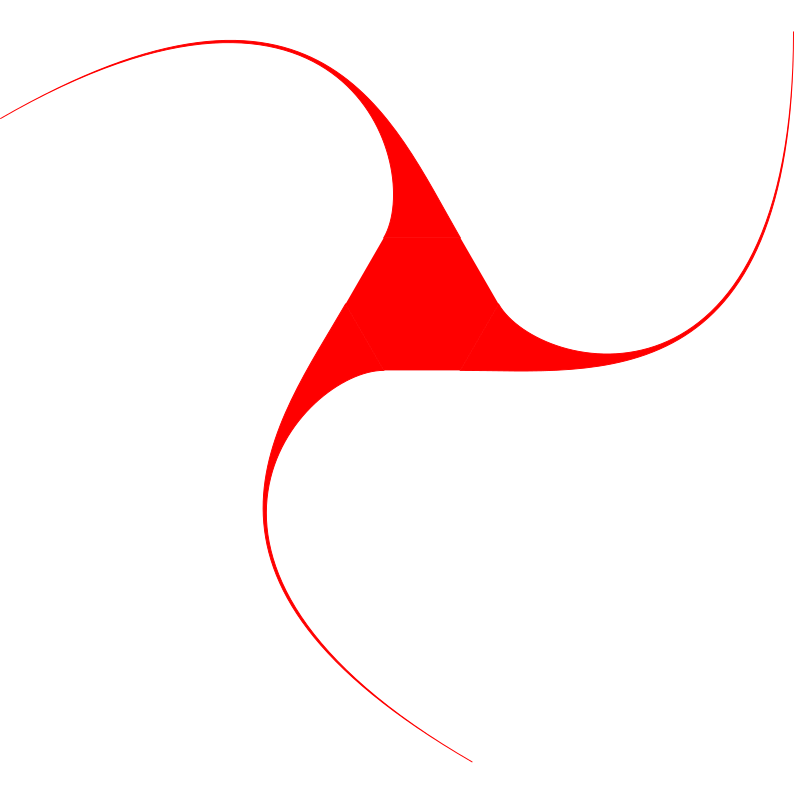# Symmetry#

class orix.quaternion.Symmetry(data: )[source]#

Bases: Rotation

The set of rotations comprising a point group.

An object’s symmetry can be characterized by the transformations relating symmetrically-equivalent views on that object. Consider the following shape.This obviously has three-fold symmetry. If we rotated it by $$\frac{2}{3}\pi$$ or $$\frac{4}{3}\pi$$, the image would be unchanged. These angles, as well as $$0$$, or the identity, expressed as quaternions, form a group. Applying any operation in the group to any other results in another member of the group.

Symmetries can consist of rotations or inversions, expressed as improper rotations. A mirror symmetry is equivalent to a 2-fold rotation combined with inversion.

Attributes

 Symmetry.contains_inversion Return whether this group contains inversion. Symmetry.diads Return the diads of this symmetry. Symmetry.euler_fundamental_region Return the fundamental Euler angle region of the proper subgroup. Symmetry.fundamental_sector Return the fundamental sector describing the inverse pole figure given by the point group name. Symmetry.is_proper Return whether this group contains only proper rotations. Symmetry.laue Return this group plus inversion. Symmetry.laue_proper_subgroup Return the proper subgroup of this group plus inversion. Symmetry.name Symmetry.order Return the number of elements of the group. Symmetry.proper_subgroup Return the largest proper group of this subgroup. Symmetry.proper_subgroups Return the list of proper groups that are subgroups of this group. Symmetry.subgroups Return the list groups that are subgroups of this group. Symmetry.system Return which of the seven crystal systems this symmetry belongs to.

Methods

 Symmetry.from_generators(*generators) Create a Symmetry from a minimum list of generating transformations. Symmetry.plot([orientation, ...]) Stereographic projection of symmetry operations.